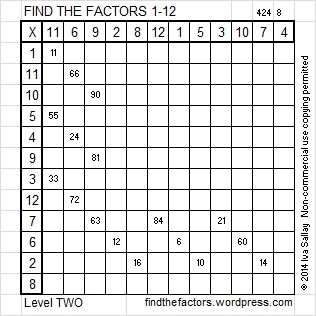# 149 and Level 2

• 149 is a prime number.
• Prime factorization: 149 is prime.
• The exponent of prime number 149 is 1. Adding 1 to that exponent we get (1 + 1) = 2. Therefore 149 has exactly 2 factors.
• Factors of 149: 1, 149
• Factor pairs: 149 = 1 x 149
• 149 has no square factors that allow its square root to be simplified. √149 ≈ 12.2065556How do we know that 149 is a prime number? If 149 were not a prime number, then it would be divisible by at least one prime number less than or equal to √149 ≈ 12.2. Since 149 cannot be divided evenly by 2, 3, 5, 7, or 11, we know that 149 is a prime number.Excel file of puzzles and previous week’s factor solutions: 12 Factors 2014-06-16This site uses Akismet to reduce spam. Learn how your comment data is processed.